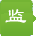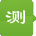## 监测人关于混沌时间序列讲座（1）：互信息法求延迟时间

1、混沌理论

2、互信息法求延迟时间

在进行重构相空间时，延迟时间和嵌入维数的选取具有十分重要的意义，同时这两个参数确定也是难点。相关基础理论知识，监测人网站此次讲座就不在此一一细细讲解，具体可以查看吕金虎编写的《混沌时间序列分析及其应用》，里面有较为详细的介绍。

计算延迟时间的方法主要有自相关函数法、复自相关法、互信息法，其中互信息法是估计重构相空间时间延迟的一种有效方法，得到了广泛的应用。因此，本次讲座主要讲述关于互信息法求延迟时间的相关知识。

function [tau,I_sq]=mutual_information(data,tau_max,n)

I_sq=zeros(tau_max,1);

N=length(data);

for tau=1:tau_max

s=data(1:N-tau);q=data(tau+1:N);

as=min(s);bq=min(q);    delts=(max(s)-as)/n;deltq=(max(q)-bq)/n;

N_sq=zeros(n);

for ii=1:n

for jj=1:n

for k=1:N-tau

as_k=(s(k)-as)/delts; bq_k=(q(k)-bq)/deltq;

if as_k>=ii-1&as_k<ii&bq_k>=jj-1&bq_k<jj

N_sq(ii,jj)=N_sq(ii,jj)+1;

end

end

end

end

Ntotal=sum(sum(N_sq));

Ps=sum(N_sq)/Ntotal;   %¼ÆËãÎ»ÓÚÒ»Î¬s¸ñ×ÓÄÚµÄ¸ÅÂÊ

Pq=sum(N_sq')/Ntotal;  %¼ÆËãÎ»ÓÚÒ»Î¬q¸ñ×ÓÄÚµÄ¸ÅÂÊ

Psq=N_sq/Ntotal;       %¼ÆËãÎ»ÓÚ¶þÎ¬¸ñ×Ó(ii,jj)ÄÚ¸ÅÂÊ

H_s=0; %¼ÆËãsµÄìØ

H_q=0; %¼ÆËãqµÄìØ

for i=1:n

if Ps(i)~=0

H_s=H_s-Ps(i)*log(Ps(i));

elseif Pq(i)~=0

H_q=H_q-Pq(i)*log(Pq(i));

end

end

(12)
100%

(0)
0%
------分隔线----------------------------

•••••••监测人 用户等级:注册会员 注册时间:1970-01-01 08:01 最后登录:2021-08-12 14:08